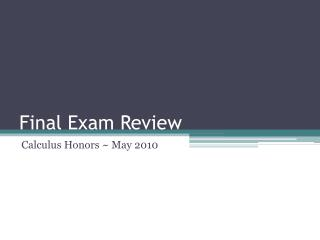DownloadDownload PresentationFinal Exam Review

# Final Exam Review

Download Presentation## Final Exam Review

- - - - - - - - - - - - - - - - - - - - - - - - - - - E N D - - - - - - - - - - - - - - - - - - - - - - - - - - -
##### Presentation Transcript

1. Final Exam Review Calculus Honors ~ May 2010

2. Construction of Exam • 10 True-False • 7 Multiple Choice • 11 Open Response (I take the best 10) • 1 sheet of 8.5 x 11in. notes is allowed (you do not need your table of integral values for this test) • Graphing Calculator allowed during the whole test

3. Topics to Consider • when is a graph continuous • graphing with relative extrema, increasing/decreasing, concavity • acceleration, velocity, position • know your trig derivatives • Chain Rule!! • Integrals – bounded and unbounded • Do you know how to use your calculator to find the area under the curve? • profit, revenue, cost • equation of a line tangent to a graph at a point • find absolute max/min values over the real line or intervals • taking the 1st derivative, 2ndder, 3rd der. • limits • using your graphing calculator to find integral • Finding - look at your old test for notes with this. • initial value problems • How do basic graphs shift? • simplified difference quotient formula and how to use it • area under the curve

4. Sketch the function showing the following:a. Interceptsb. Asymptotesc. Undefined values and critical pointsd. Increasing or decreasing extremae. Inflection pointsf. Concavityg. Sketch

5. Profit, Revenue and Cost Show profit function. How many units must be produced to maximize profit? What is the maximum profit?

6. Tangent Lines • Find the equation of a line tangent to the graph at the point (1,4).

7. Absolute Max/Min Problem • Find the absolute max/min value over the indicated interval or the real number line.

8. Derivatives

9. Limits Find the following limits if they exist:

10. Find the simplified difference quotient for the following: